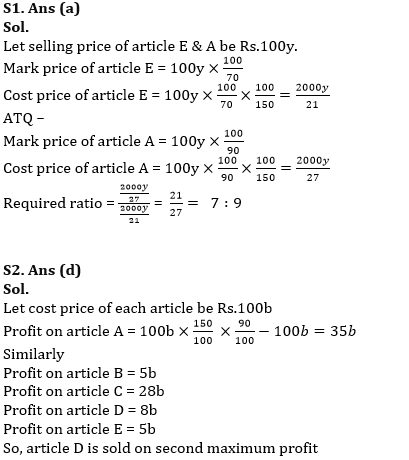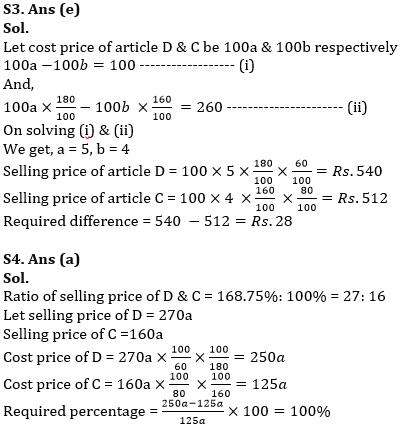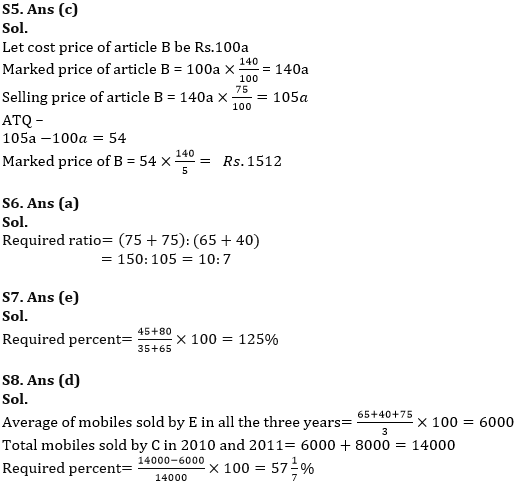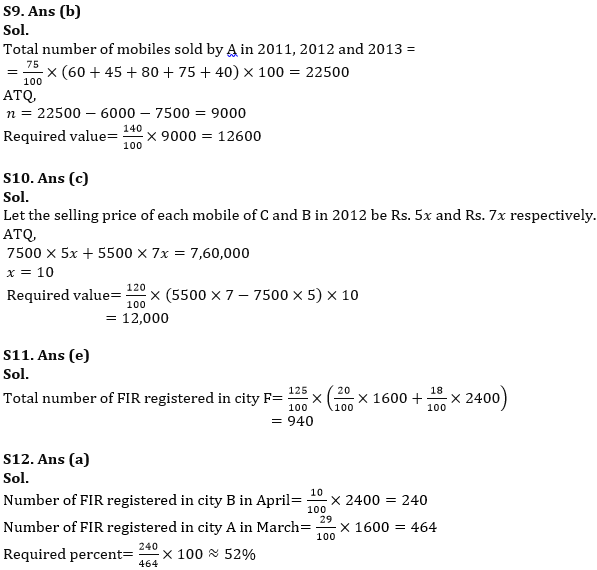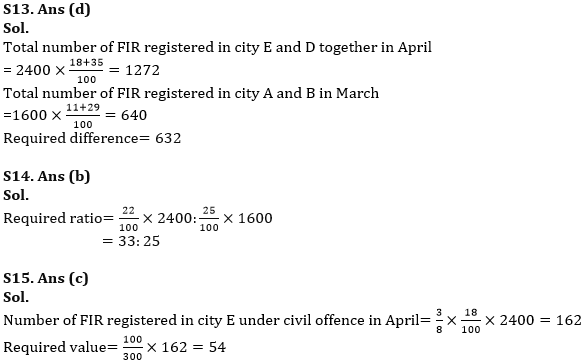Latest Banking jobs   »   Quantitative Aptitude Quiz For RBI Grade...

# Quantitative Aptitude Quiz For RBI Grade B/ ECGC PO/ SIDBI Grade A Prelims 2022- 26thApril

Directions (1–5): Bar graph given below shows markup percentage and discount percentage on five different articles sold by a shopkeeper. Study the data carefully and answer the following questions.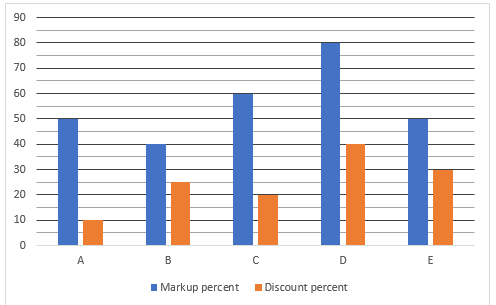Q1. If the ratio of selling price of article E to that of article A is 1 : 1, then find out the respective ratio of cost price of article A to that of article E?
(a)7:9
(b)9:7
(c)9:8
(d)8:9
(e)None of these

Q2. If cost price of each article is same, then which article is sold at third maximum profit?
(a)A
(b)B
(c)C
(d)D
(e)E

Q3. If the cost price of article D is Rs.100 more than cost price of article C, while mark price of article D is Rs.260 more than mark price of article C, then find the difference between selling price of article D and that of article C?
(a)54
(b)48
(c)42
(d)36
(e)28

Q4. If the selling price of article D is 68 ¾ % more than that of article C, then find the cost price of article D is what percent more/less than that of article C?
(a)100%
(b)50%
(c)150%
(d)75%
(e)125%

Q5. If shopkeeper earn Rs.54 profit on selling article B, then find the Mark price of article B.
(a)None of these
(b)1620
(c)1512
(d)1718
(e)1416

Directions (6-10): Bar graph shows the number of mobiles (in hundred) sold by five different companies (A, B, C, D & E) in three different years (2010, 2011 & 2012). Study the bar graph carefully and answer the following questions.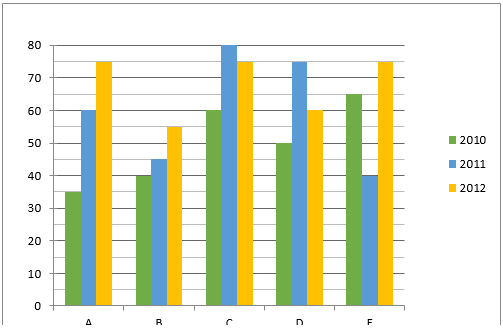Q6. Find the respective ratio of total mobiles sold by A & C together in 2012 to total mobiles sold by E in 2011 & 2010 together?
(a)10:7
(b)7:9
(c)9:8
(d)11:10
(e)9:10

Q7. Find the total mobiles sold by B & C together in 2011 are what percent of total mobiles sold by A & E together in 2010?
(a)200%
(b)150%
(c)50%
(d)75%
(e)125%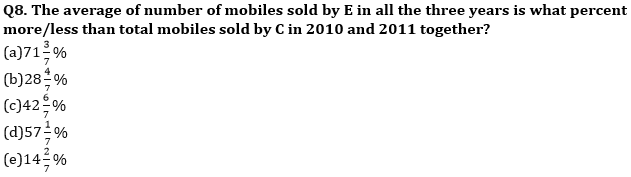Q9. If n is the number of mobiles sold by A on 2013 and the total number of mobiles sold by A in 2011, 2012 and 2013 together is 75% of total mobiles sold by all five companies together in 2011, then find the value of 140% of n?
(a)14800
(b)12600
(c)17200
(d)18800
(e)11200

Q10. If the selling price of each mobile sold in 2012 of C to that of B is 5: 7 and sum of revenue generated from both of them in 2012 is Rs.7,60,000, then find value of 120% of difference in revenue generated from mobiles sold in 2012 of B and C?
(a) None of these
(b) Rs.22000
(c) Rs.12000
(d) Rs.16000
(e) Rs.26000

Directions (11-15): Read the following line graph carefully and answer the questions given below.
The line graph shows percentage distribution of no. of FIR registered in five different cities in two different months.
Note: Total FIR registered in March=1600
Total FIR registered in April=2400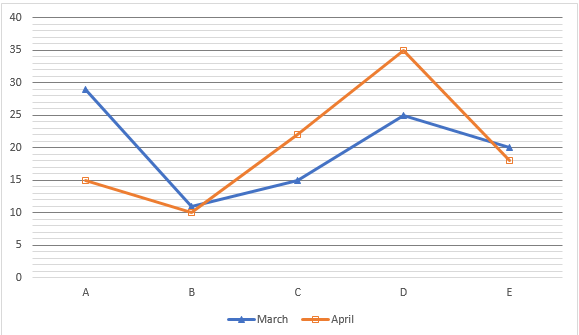Q11. If the number of FIR’s registered in city F in both the months together is 25% more than number of FIR’s registered in city E in both the months together, then find the total number of FIR’s registered in city F in both the month together?
(a)900
(b)980
(c)840
(d)880
(e)940

Q12. Find the number of FIR’s registered in city B in April is approximately what percent of number FIR’s registered in city A in March?
(a)52%
(b)56%
(c)46%
(d)42%
(e)60%

Q13. Find the total number of FIR’s registered in city E and D together in April is how much more/less than total number of FIR’s registered in city A and B in March?
(a)None of these
(b)756
(c)696
(d)632
(e)712

Q14. Find the respective ratio of total number of FIR’s registered in city C in April to total number of FIR’s registered in city D in March?
(a)25:34
(b)33:25
(c)29:31
(d)31:20
(e)29:25

Q15. If the ratio of FIR registered in city E under civil to criminal offence is 3:5 in the month of April, then find the value of 33⅓% of FIR registered under civil offence?
(a)36
(b)42
(c)54
(d)62
(e)80

Solutions